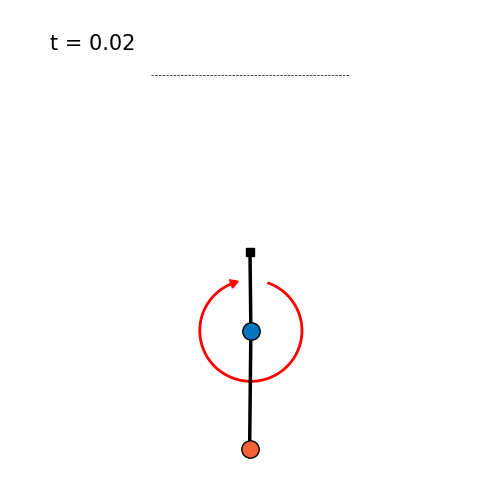Controller Short Controller Description Swingup Success Swingup Time [s] Energy [J] Max. Torque [Nm] Integrated Torque [Nms] Torque Cost [N²m²] Torque Smoothness [Nm] Velocity Cost [m²/s²] RealAI Score Username Data
iLQR MPC stabilization Online optimization with iterative LQR. Stabilization of iLQR trajectory. Top stabilization with LQR. 1/1 4.15 10.8 4.47 2.61 2.34 0.076 99.12 0.806 fwiebe data plot video
TVLQR Stabilization of iLQR trajectory with time-varying LQR. 1/1 3.98 10.92 5.0 2.27 2.47 0.077 100.34 0.8 fwiebe data plot video
SAC LQR Swing-up with an RL Policy learned with SAC. 1/1 1.55 24.86 4.98 3.72 10.32 0.558 158.35 0.811 chiniklas data plot video
iLQR Riccati Gains Stabilization of iLQR trajectorry with Riccati gains. Top stabilizaion with LQR. 1/1 4.15 10.69 3.01 2.35 2.2 0.013 99.16 0.831 fwiebe data plot video
mcpilco Swingup trained with MBRL algorithm MC-PILCO + stabilization with LQR. 1/1 1.1 9.81 2.82 1.27 2.27 0.057 242.44 0.869 turcato-niccolo data plot video
Energy PFL Partial Feedback Linearization with energy shaping control. Stabilization with LQR. 1/1 4.12 29.01 5.0 7.12 18.79 0.251 280.81 0.728 fwiebe data plot video
iLQR MPC Online optimization with iterative LQR. Without reference trajectory. 1/1 1.47 29.62 6.0 3.47 12.16 0.046 175.52 0.796 fwiebe data plot video

## Rules

The simulation leaderboard compares the performance of different control methods in simulation. The task for the controller is to swingup and balance the acrobot and keep the end-effector above the threshold line.The model parameters of the acrobot are:

• Gravity: $$g = 9.81 \, \frac{\text{m}}{\text{s}^2}$$
• First Link mass: $$m_1 = 0.6416937799349799 \, \text{kg}$$
• Second Link mass: $$m_2 = 0.563935954099688 \, \text{kg}$$
• First actuator length: $$l_1 = 0.2 \, \text{m}$$
• Second actuator length: $$l_2 = 0.3 \, \text{m}$$
• First link center of mass: $$r_1 = 0.2 \, \text{m}$$
• Second link center of mass: $$r_2: 0.3212669909674605 \, \text{m}$$
• First link inertia: $$I_1 = 0.02671076499939512 \, \text{kg m}^2$$
• Second link inertia: $$I_2 = 0.053878129629650884 \, \text{kg m}^2$$
• First actuator damping friction: $$b_1 = 0.0 \, \frac{\text{kg m}}{s}$$
• Second actuator damping friction: $$b_2 = 0.0 \, \frac{\text{kg m}}{s}$$
• First actuator coulomb friction: $$c_{f1}: 0.0 \, \text{N m}$$
• Second actuator coulomb friction: $$c_{f2}: 0.0 \, \text{N m}$$
• Motor Inertia: $$I_r = 0.0 \, \text{kg m}^2$$
• Gear ratio: $$g_r: 6.0$$
• First Motor Torque limit $$\tau_{max1} = 0.0 \, \text{N m}$$
• Second Motor Torque limit $$\tau_{max2} = 6.0 \, \text{N m}$$

More information about the dynamic model of the double pendulum can be found here: Double Pendulum Dynamics. For a urdf file with this model see here: URDF.

The acrobot is simulated with a Runge-Kutta 4 integrator with a timestep of $$dt = 0.002 \, \text{s}$$ for $$T = 10 \, \text{s}$$. The initial acrobot configuration is $$x_0 = (0, 0, 0, 0)$$ (hanging down) and the goal is the unstable fixpoint at the upright configuration $$x_g = (\pi, 0, 0, 0)$$. The upright position is considered to be reached when the end-effector is above the threshold line at $$h=0.45 \, \text{m}$$ (origin at the mounting point).

## Scores

For the evaluation multiple criteria are evaluated and weighted to calculate an overall score (Real AI Score). The criteria are:

• Swingup Success $$c_{success}$$: Whether the swingup was successful, i.e. if the end-effector is above the threshold line in the end of the simulation.
• Swingup time $$c_{time}$$: The time it takes for the acrobot to reach the goal region above the threhhold line and stay there. If the end-effector enters the goal region but falls below the line before the simulation time is over the swingup is not considered successful! The swingup time is the time when the end-effector enters the goal region and does not leave the region until the end.
• Energy $$c_{energy}$$: The mechanical energy used during the execution.
• Max Torque $$c_{\tau, max}$$: The peak torque that was used during the execution.
• Integrated Torque $$c_{\tau, integ}$$: The time integral over the used torque over the execution duration.
• Torque Cost $$c_{\tau, cost}$$: A quadratic cost on the used torques ( $$c_{\tau, cost} = \sum u^TRu$$, with R = 1).
• Torque Smoothness $$c_{\tau, smooth}$$: The standard deviation of the changes in the torque signal.
• Velocity Cost $$c_{vel, cost}$$: A quadratic cost on the joint velocities that were reached during the execution ( $$c_{vel} = \dot{q}^T Q \dot{q}$$, with Q = identity)

These criteria are used to calculate the overall Real AI Score with the formula

$\begin{equation} S = c_{success} \left( w_{time}\frac{c_{time}}{n_{time}} + w_{energy}\frac{c_{energy}}{n_{energy}} + w_{\tau, max}\frac{c_{\tau, max}}{n_{\tau, max}} + w_{\tau, integ}\frac{c_{\tau, integ}}{n_{\tau, integ}} + w_{\tau, cost}\frac{c_{\tau, cost}}{n_{\tau, cost}} + w_{\tau, smooth}\frac{c_{\tau, smooth}}{n_{\tau, smooth}} + w_{vel, cost}\frac{c_{vel, cost}}{n_{vel, cost}} \right) \end{equation}$

The weights and normalizations are:

Criterion normalization $$n$$ weight $$w$$
Swingup Time 10.0 0.2
Energy 100.0 0.1
Max Torque 6.0 0.1
Integrated Torque 60.0 0.1
Torque Cost 360 0.1
Torque Smoothness 12.0 0.2
Velocity Cost 1000.0 0.2

## Participating

If you want to participate in this leaderboard with your own controller have a look at the leaderboard explanation in the double pendulum repository. The leaderboard is automatically periodically updated based on the controllers that have been contributed to that repository.